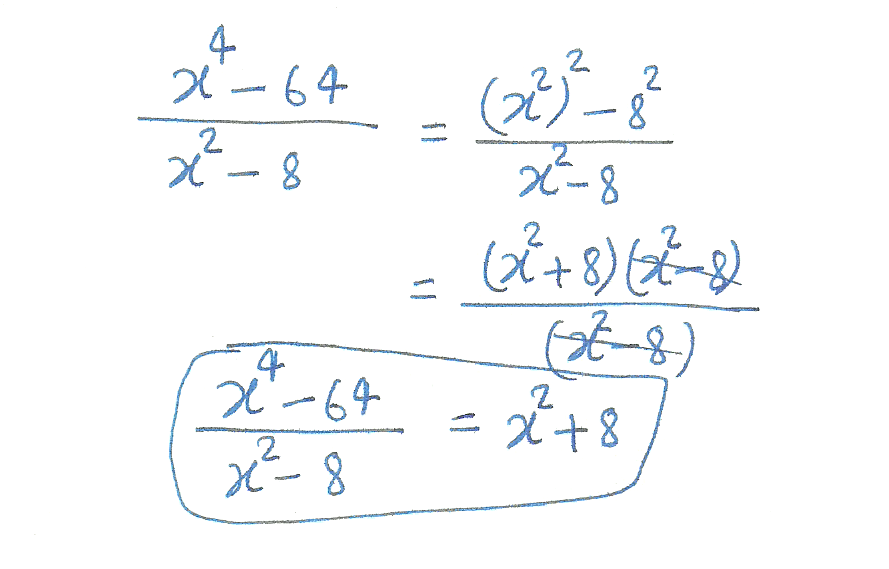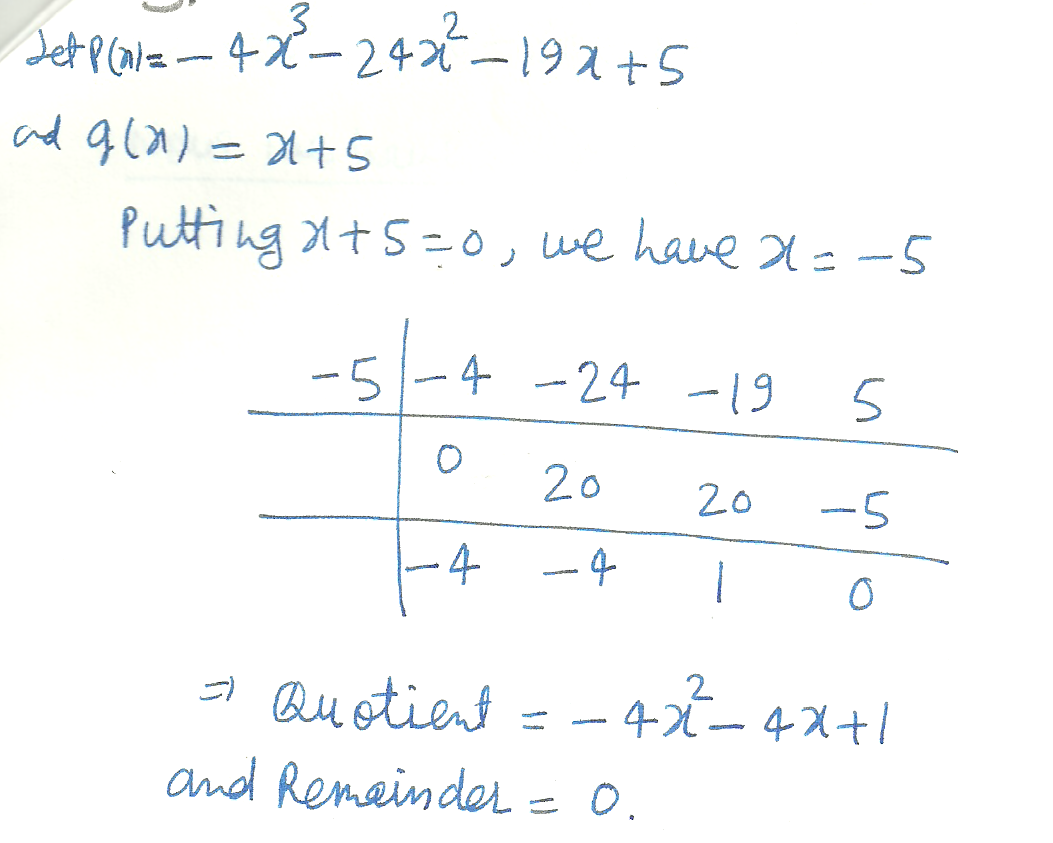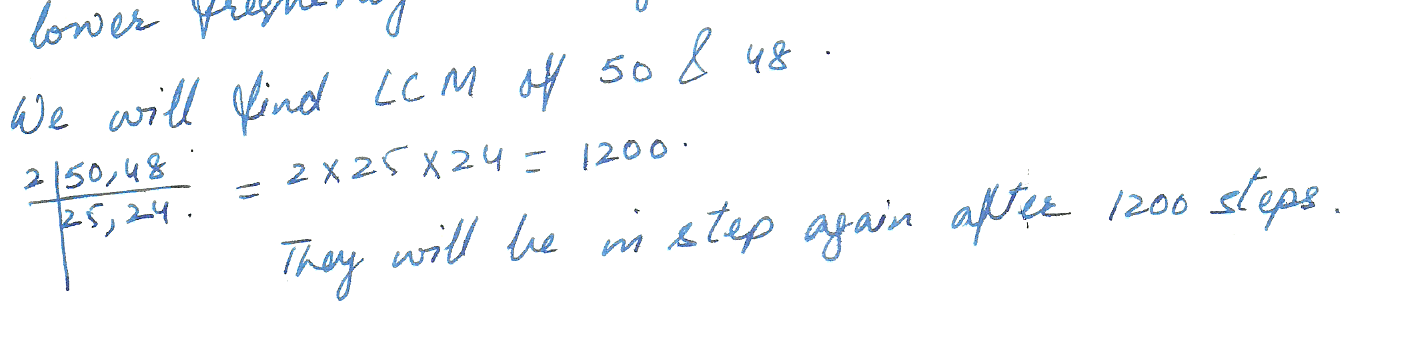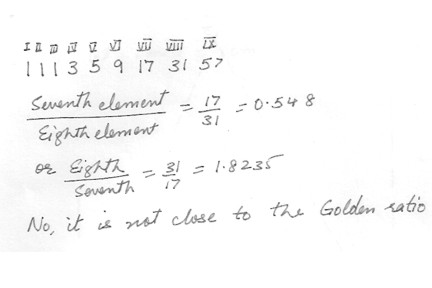4375

Mathematics Algebra Level: Misc Level

solve the problem ( x ^ 4 - 64 )divided by ( x^2 -8 )4374

Mathematics Algebra Level: Misc Level

use synthetic division to determine the quotient and remainder,

(- 4 x^ 3 - 24 x^ 2 - 19 x + 5 ) divided by ( x + 5 )4349

Mathematics Algebra Level: Misc Level

Walking beside you, your friend takes 50 strides per minute while you take 48 strides per minute. If you start in step, when will you be in step again?3602

Mathematics Algebra Level: University

Events A  B and C are mutually independent events with P(A)=.3  P(B)=.4  and P(C)=.5.  What is the probability that:

a) Exactly two of them happen
b) At least two of them happen
c) Not more than two of them happen3598

Mathematics Algebra Level: University

Events A, B, and C are mutually independent events with P(A)=.3, P(B)=.4, and P(C)=.5.  What is the probability that:

a) Exactly two of them happen
b) At least two of them happen
c) Not more than two of them happen
##### Question Diagram3514

Mathematics Algebra Level: University

Draw the graph for the following linear equations.

Y= X + 7

Y=1 X - 6
53513

Mathematics Algebra Level: University

Draw the graph for the following linear equation.
Y + 4X =83512

Mathematics Algebra Level: University

Draw the graph for the following linear equation.

x + 2y =33193

Mathematics Algebra Level: University

Do you think the current highway speed in Canada is appropriate? How would you decide whether or not it should be increased or decreased?

Focus: Applying the marginalist principle to try to find an optimal speed limit for road traffic.341

Mathematics Algebra Level: High School

Consider the sequence that begins 1,1,1… and continues by the rule: Every subsequent element of the sequence is the sum of the three preceding elements. Determine the next five (fourth through eighth) elements of the sequence. Compute the ration of the seventh and eighth elements of the sequence. Is it close to the Golden Ratio ?Displaying 31-40 of 40 results.